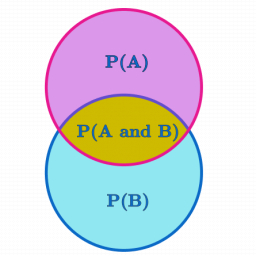# Students and exam

In a certain college, accounting is one of the courses; among the accounting students, 60% are male. Among the male students, 75% passed the exams, while among the females, 50% failed.
(a) present this using a probability tree diagram
(b) determine the probability that an accounting student selected at random passed the exams
(c)an accounting student is selected at random. Find the probability that it is a male
(d)an accounting student who failed the exam. Determine she is female

b =  0.65
c =  0.6
d =  0.875

### Step-by-step explanation:Did you find an error or inaccuracy? Feel free to write us. Thank you!

Tips for related online calculators
Need help calculating sum, simplifying, or multiplying fractions? Try our fraction calculator.